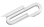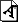Spring 2020

# Tue April 7

First, discuss the linear regression assignment, and answer any questions.

Note that the notation I used in the last few notebooks isn't (in spite of my intentions) the same as the textbook. I was using y = a * x + b while the book was using y = b * x + a. Go figure. Just make sure that whatever notation and variables you are using is internally consistent.

# in class

We decided not to any of the things I had described below, but instead pivot towards neural nets and deep learning for the next few weeks.

Our textbook takes a look at neural networks and deep learning in chapters 18 and 19 - and even uses those names.

So read chapter 18 for Friday, and we'll discuss that material then.

# Next

Several choices ... and start thinking about final projects.

## choice 1 : multi linear regression

• multiple columns i.e. "multi linear"
• add extra terms to error function to penalize more variables; this is called "regularization"
• ridge regression : if using $$y = a_1 x_1 + a_2 x_2 + b$$ , add $$γ * (a_1*a_1 + a_2*a_2)$$ to error where γ is an arbitrary constant.
• lasso regression : add $$γ * |a_1| + |a_2|$$ instead.

Note that the book is using symbolic calculus to find an analytic formula for the gradient, rather than just calculating it numerically as I did in my last worksheet. My way is simpler conceptually and always works, but may well be too computationally expensive.

Also note that when we says the lasso cannot be used with gradient descent, what he really means is that you can't find an analytic derivative. I'm not convinced that gradient descent itself, the way I set it up with differences, doesn't work at all.

Gradient descent - and other machine learning methods - are also often done using randomly chosen subsets of the training data for each step in looking for the model parameters. You can even randomly choose a single row of the training data and use that to improve the model parameters a little bit, and then repeat many times. Why use less data? Because the calculation will be faster ... even though the step will be less accurate.

Your possible-mission-number-1 is to play with a multiple linear regression model. All the details of which variation are up to you.

I suggest you re-use the data you used before for the nearest neighbor model, and see if multi-linear regression does better or worse.

Or use a different one of of the datasets we used for nearest neighbors .

Or use one of these :

## choice 2 : think about COVID-19 modeling

... since it seems like that's all anyone can talk about right now.

And modeling is certainly part of data science.

So ... here are a few places to start reading, if that's what we want to do.

If there's interest, we could talk about "difference equations" as opposed to "differential equations", what that has to do with modeling, and perhaps set up a toy exponential growth website with a graph.

"Modeling" often (though not always) means simulating something over time. It's writing down some differential (or difference) equations, solving them numerically, and then comparing with what you know from the data. Then adjust and repeat.

For background on this (huge) topic, see for example

## final projects

AND don't forget that you should be thinking about some dataset and/or problem that you yourself would like to investigate numerically as a final project.

I will make picking something part of next Tuesday's assignment, and would like you to be ready to talk about that then - both what you have in mind, and what dataset you're going to use.

We'll do final project presentations the last day of classes - Tue May 5 - that's a month away.

https://cs.marlboro.college /cours /spring2020 /data /notes /apr7
## attachmentslast modified sizeexponential_growth.pdf Thu Jul 16 2020 03:39 am 122K